## Resource of the week: Money and place value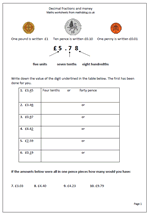Money is a great way to reinforce place value with decimals. For example in the total £3.45 the digit 4 has a value of 4 tenths or 40p. Money makes it very easy to show tenths (a 10p coin is one tenth of a pound) and hundredths (a one pence coin is one hundredth of a pound).

This page looks at this in more detail, showing that ten pence can be written in two ways; either as 10p or as part of a pound £0.10. There are two further things to remember here:

1. that we should always have two digits after the decimal point when writing fractions of a pound eg £0.30 not £0.3.

2. that we should not include both the pound and pence sign when writing amounts in pounds eg £0.30 is correct but £0.30p is not. it is 0.30 of a pound, not 0.30 of a penny.

This is just one worksheet from a great selection in our Counting and Understanding Numbers section within our year 4 maths worksheets. Why not go and have a look at what else is freely available?

Decimal fractions and money

## More year 4 measurement word problems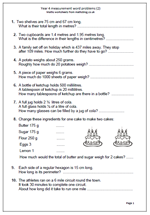Following the publication of our first set of measurement word problems for year 4 we have had several requests for some more: so here is the second page.

The questions cover a range of measurements, including converting centimetres to metres  and working with millilitres, grams and even miles. Space is given to write the answers, although children may need to make jottings or even use standard written methods to calculate some of the answers.

Most of these questions are ‘one-step’ in that only one maths operation needs to be done to find the answer, which does make them slightly easier, although children do seem to struggle with word problems generally.

Measuring word problems (2)

## Resource of the Week: Year 2 symmetry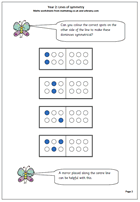Folding to create symmetrical patterns is a popular past-time in schools, but understanding reflective symmetry can be quite tricky. These maths worksheets for year 2  ask children to colour the correct spots on the other side of the line of symmetry to make sure that the shape is symmetrical.

Pegboards are a useful aid to this work, as is a mirror, which can be laid along the line, or axis of symmetry to see how the pattern continues. Watch out for children who just copy the image rather than show the reflection.

More can be found in our year 2, Understanding shape section.

Symmetry_1

## A Maths Challenge: 12 to 15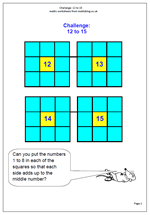I have not put up many challenges and investigations recently so I thought I would redress this over the coming weeks with some fun and challenging tasks. Using and Applying Maths has been a cornerstone of the Primary Framework for Mathematics and these kinds of activities are important in making children think through a problem and work in a logical, well organised way.

This challenge is actually in four parts, but one the first has been cracked the same method can be applied to the others.  A 3 by 3 square grid has the number 12 in the middle. The challenge is to place each of the digits from 1 to 8 around the grid so that each side across and down adds up to the middle number (12).

A really good way to approach this task is to cut out the numbers from 1 to 8 so that they can easily be moved around the grid. There are several different solutions to the task, but they all have a similar pattern.

Challenge 12 to 15

## Resource of the Week: percentagesThis week’s resource of the Week highlights the second of our percentages worksheets for Year 5. It is typical of the English that we manage to write per cent in two different ways. Unlike the Americans who use percent we use two words per cent, apart from when we write percentage when we put it altogether.Anyway, as to the maths: remember that many percentages can be worked out ‘in your head’ by remembering that 10% of an amount is equivalent to dividing the amount by 10. At this stage we will only be dealing with whole tens so it is probably the best approach. Later more complicated percentages will be found by dividing by 100 and multiplying by the percentage.

To find 20% of £350.

Find 10% of £350, which  is £35

If 10% is £35, then 20% will be £35 x 2 = £70.

Percentages (pg 2)

## Using a number square in year 2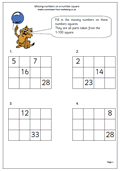Here we have one page from a selection found in Year 2 which looks in detail at a 1-100 number square and is excellent practice at understanding place value as well as counting. We often take it for granted that children can count up to 100 easily, but in fact many children are not at all confident with this.

A small section of the whole number square, just 4 by 3 is taken and most of the numbers removed. The skill is in replacing the missing numbers correctly.

This can be done in a variety of ways. Probably the easiest way is to work across as each number is one more than the number to the left. One of the more interesting ways is to work down as each number is 10 more than the number above.

Missing numbers on a number square (pg 2)

## Resource of the week: Valentine’s Day Maths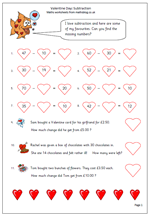Looking through the range of resources it occurred to me that Valentine’s Day is coming up shortly. Sometimes it’s nice to have a change and special occasions such as Valentine’s Day allows us to do this. Here we have a maths worksheet suitable for Year 3 children with a Valentine Day’s theme. It is a subtraction page but all the missing numbers have to be put into the special Valentine hearts. There are also a couple of Valentine word problems.

The easiest way to do most of these subtractions is by adding on from the smaller number, once again showing the special relationship between addition and subtraction.

Thanks to urbrainy.com for letting me use this page from their vast supply of worksheets, including more on Valentine’s Day.

Valentine subtraction

## Year 6 money problems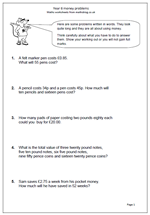There are not many questions on this page but they are tricky with some requiring several stages to answer. They are all to do with money and involve addition, multiplication and division.

As a preview to answering SAT questions this page asks for all working out to be shown and tips on the answer page will help decide whether an extra mark can be given for showing suitable working out, even if the final answer is incorrect. (Be very suspicious of any answers which are written down without showing any working as they may well have been answered using a calculator.)

Money problems